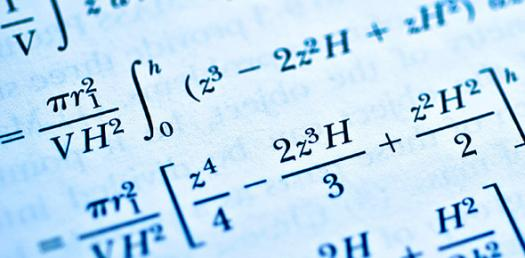Example Of A Statistic Quiz Questions

15 Questions | Total Attempts: 2264Settings.

• 1.
The range of the data:25, 18, 20, 22, 16, 6, 17, 12, 30, 32, 10, 19, 8, 11, 20 is
• A.

10

• B.

15

• C.

18

• D.

26

• 2.
In the class interval 10-20, 20-30, the number 20 is included in:
• A.

10-20

• B.

20-30

• C.

Both the intervals

• D.

None of these intervals

• 3.
To draw a histogram to represent the following frequency distribution: Class interval5-1010-1515-2525-4545-15Frequency61210815the adjusted frequency for the class 25-45 is:
• A.

6

• B.

5

• C.

3

• D.

2

• 4.
A grouped frequency distribution table with classes of equal sizes using;63-72 (72 included) as one  of the class is constructed for the following data:30, 32, 45, 54, 74, 78, 108, 112, 66, 76, 88,40, 14, 20, 15, 35, 44, 66, 75, 84, 95, 96,102, 110, 88, 74, 112, 14, 34, 44The number of classes in the distribution will be:
• A.

9

• B.

10

• C.

11

• D.

12

• 5.
The mean of five numbers is 30. If one number is excluded,  their mean becomes 28. The excluded number is:
• A.

28

• B.

30

• C.

35

• D.

38

• 6.
There are 50 numbers. Each number is subtracted from 53 and the mean of the numbers so obtained is found to be –3.5. The mean of the given numbers is:
• A.

46.5

• B.

49.5

• C.

53.5

• D.

56.5

• 7.
The mean factors of 24 is:
• A.
• B.
• C.
• D.
• 8.
If the mean of the observations:x, x + 3, x + 5, x + 7, x + 10is 9, the mean of the last three observations is:
• A.
• B.
• C.
• D.
• 9.
The range of the data 25.7, 16.3, 2.8, 21.7, 24.3, 22.7, 24.9 is:
• A.

22

• B.

22.9

• C.

21.7

• D.

20.5

• 10.
If the class marks in a frequency distribution are 19.2, 26.5, 33.5, 40.5, then thye class corresponding to the class mark 33.5 is:
• A.

16-23

• B.

23-30

• C.

30-37

• D.

37-41

• 11.
The mean wage of 150 labourers working in a factory running three shifts with 60, 40 and 50 labourers is Rs. 114. The mean wage of 60 labourers in the first shift is Rs.. 121.50 and that of 40 labourers working the second shift is Rs. 107.75, then the mean wage of those working in the third shift is:
• A.

Rs. 100

• B.

Rs. 110

• C.

Rs. 115.75

• D.

Rs. 120

• 12.
The number of times a particular items occur in a class interval called its:
• A.

Mean

• B.

Frequency

• C.

Cumulative frequency

• D.

Range

• 13.
Let m be the mid-point and l be the upper class limit of a class in a continuous frequency distribution. The lower class limit of the class is:
• A.

2m + l

• B.

2m – l

• C.

M – l

• D.

M – 2l

• 14.
Kavita obtained 16, 14, 18 and 20 marks (out of 25) in Maths in weekly tests in the month of Jan. 2000; then meanh marks of Kavita is:
• A.

16

• B.

16.5

• C.

17

• D.

17.5

• 15.
The width of each o five continuous classes in a frequency distribution is 5 and lower class-limit of the lowest class is 10. The upper class-limit of the highest class is:
• A.

15

• B.

25

• C.

35

• D.

40

Related TopicsBack to top